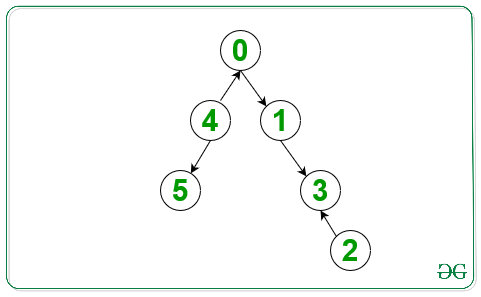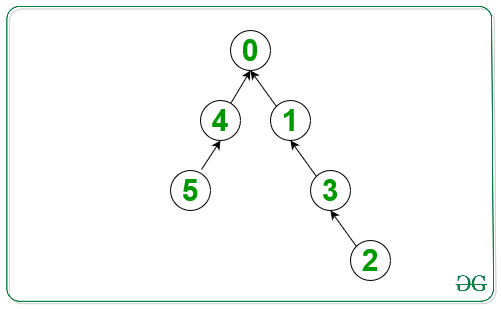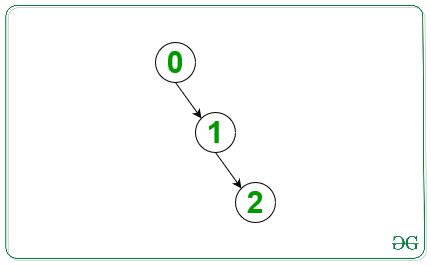Related Articles

# Minimum number of reversals to reach node 0 from every other node

• Difficulty Level : Hard
• Last Updated : 14 Dec, 2020

Given a Directed Graph with N vertices value from 0 to N – 1 and N – 1 Edges, the task is to count the number of edges must be reversed so that there is always a path from every node to node 0.

Examples:

Attention reader! Don’t stop learning now. Get hold of all the important DSA concepts with the DSA Self Paced Course at a student-friendly price and become industry ready.  To complete your preparation from learning a language to DS Algo and many more,  please refer Complete Interview Preparation Course.

In case you wish to attend live classes with experts, please refer DSA Live Classes for Working Professionals and Competitive Programming Live for Students.

Input: Below is the given graphOutput:
Explanation:Input: Below is the given graphOutput: 0

Approach: The idea is to use BFS Traversal for a graph. Below are the steps:

1. Create a directed graph with the direction of the edges of the given graph reversed.
2. Create a queue and push node 0 in the queue.
3. During BFS Traversal on the graph do the following:
• Pop the front node(say current_node) from the queue.
• Traverse the Adjacency List of current node in the reverse graph and push in those node in the queue that are not visited.
• Traverse the Adjacency List of current node in the reverse graph and push in those node in the queue that are not visited.
• The total nodes inserted in the queue in the above steps is required count of edges to be reversed because the nodes which are connected to the current node and have not been visited yet in the graph cannot be reached to node 0, therefore, we need to reverse their direction. Add the count of the nodes in the above step to the final count.

Below is the implementation of the above approach:

## C++

 `// C++ program for the above approach``#include ``using` `namespace` `std;` `// Function to find minimum reversals``int` `minRev(vector > edges,``           ``int` `n)``{` `    ``// Add all adjacent nodes to``    ``// the node in the graph``    ``unordered_map<``int``, vector<``int``> > graph;``    ``unordered_map<``int``, vector<``int``> > graph_rev;` `    ``for` `(``int` `i = 0;``         ``i < edges.size(); i++) {` `        ``int` `x = edges[i];``        ``int` `y = edges[i];` `        ``// Insert edges in the graph``        ``graph[x].push_back(y);` `        ``// Insert edges in the``        ``// reversed graph``        ``graph_rev[y].push_back(x);``    ``}` `    ``queue<``int``> q;` `    ``// Create array visited to mark``    ``// all the visited nodes``    ``vector<``int``> visited(n, 0);``    ``q.push(0);` `    ``// Stores the number of``    ``// edges to be reversed``    ``int` `ans = 0;` `    ``// BFS Traversal``    ``while` `(!q.empty()) {` `        ``// Pop the current node``        ``// from the queue``        ``int` `curr = q.front();` `        ``// mark the current``        ``// node visited``        ``visited[curr] = 1;` `        ``// Intitialize count of edges``        ``// need to be reversed to 0``        ``int` `count = 0;``        ``q.pop();` `        ``// Push adjacent nodes in the``        ``// reversed graph to the queue,``        ``// if not visited``        ``for` `(``int` `i = 0;``             ``i < graph_rev[curr].size();``             ``i++) {` `            ``if` `(!visited[graph_rev[curr][i]]) {``                ``q.push(graph_rev[curr][i]);``            ``}``        ``}` `        ``// Push adjacent nodes in graph``        ``// to the queue, if not visited``        ``// count the number of``        ``// nodes added to the queue``        ``for` `(``int` `i = 0;``             ``i < graph[curr].size();``             ``i++) {` `            ``if` `(!visited[graph[curr][i]]) {``                ``q.push(graph[curr][i]);``                ``count++;``            ``}``        ``}` `        ``// Update the reverse edge``        ``// to the final count``        ``ans += count;``    ``}` `    ``// Return the result``    ``return` `ans;``}` `// Driver Code``int` `main()``{``    ``vector > edges;` `    ``// Given edges to the graph``    ``edges = { { 0, 1 }, { 1, 3 }, { 2, 3 },``              ``{ 4, 0 }, { 4, 5 } };` `    ``// Number of nodes``    ``int` `n = 6;` `    ``// Function Call``    ``cout << minRev(edges, n);``    ``return` `0;``}`

## Python3

 `# Python3 program for the above approach`` ` `# Function to find minimum reversals``def` `minRev(edges, n):`` ` `    ``# Add all adjacent nodes to``    ``# the node in the graph``    ``graph ``=` `dict``()``    ``graph_rev ``=` `dict``()``    ` `    ``for` `i ``in` `range``(``len``(edges)):`` ` `        ``x ``=` `edges[i][``0``];``        ``y ``=` `edges[i][``1``];`` ` `        ``# Insert edges in the graph``        ``if` `x ``not` `in` `graph:``            ``graph[x] ``=` `[]``        ``graph[x].append(y);`` ` `        ``# Insert edges in the``        ``# reversed graph``        ``if` `y ``not` `in` `graph_rev:``            ``graph_rev[y] ``=` `[]``        ``graph_rev[y].append(x);``    ` `    ``q ``=` `[]`` ` `    ``# Create array visited to mark``    ``# all the visited nodes``    ``visited ``=` `[``0` `for` `i ``in` `range``(n)]``    ``q.append(``0``);`` ` `    ``# Stores the number of``    ``# edges to be reversed``    ``ans ``=` `0``;`` ` `    ``# BFS Traversal``    ``while` `(``len``(q) !``=` `0``):`` ` `        ``# Pop the current node``        ``# from the queue``        ``curr ``=` `q[``0``]`` ` `        ``# mark the current``        ``# node visited``        ``visited[curr] ``=` `1``;`` ` `        ``# Intitialize count of edges``        ``# need to be reversed to 0``        ``count ``=` `0``;``        ``q.pop(``0``);`` ` `        ``# Push adjacent nodes in the``        ``# reversed graph to the queue,``        ``# if not visited``        ``if` `curr ``in` `graph_rev:``            ``for` `i ``in` `range``(``len``(graph_rev[curr])):`` ` `                ``if` `(``not` `visited[graph_rev[curr][i]]):``                    ``q.append(graph_rev[curr][i]);`` ` `        ``# Push adjacent nodes in graph``        ``# to the queue, if not visited``        ``# count the number of``        ``# nodes added to the queue``        ``if` `curr ``in` `graph:``            ``for` `i ``in` `range``(``len``(graph[curr])):`` ` `                ``if` `(``not` `visited[graph[curr][i]]):``                    ``q.append(graph[curr][i]);``                    ``count ``+``=` `1`` ` `        ``# Update the reverse edge``        ``# to the final count``        ``ans ``+``=` `count;``    ` `    ``# Return the result``    ``return` `ans;`` ` `# Driver Code``if` `__name__``=``=``'__main__'``:` `    ``edges ``=` `[]`` ` `    ``# Given edges to the graph``    ``edges ``=` `[ [ ``0``, ``1` `], [ ``1``, ``3` `], [ ``2``, ``3` `],[ ``4``, ``0` `], [ ``4``, ``5` `] ];`` ` `    ``# Number of nodes``    ``n ``=` `6``;`` ` `    ``# Function Call``    ``print``(minRev(edges, n))``    ` `# This code is contributed by rutvik_56`

## C#

 `// C# program for the above approach``using` `System;``using` `System.Collections;``using` `System.Collections.Generic;` `class` `GFG{``    ` `// Function to find minimum reversals``static` `int` `minRev(ArrayList edges, ``int` `n)``{``    ` `    ``// Add all adjacent nodes to``    ``// the node in the graph``    ``Dictionary<``int``,``               ``ArrayList> graph = ``new` `Dictionary<``int``,``                                                 ``ArrayList>();``    ` `    ``Dictionary<``int``,``               ``ArrayList> graph_rev = ``new` `Dictionary<``int``,``                                                     ``ArrayList>();``    ` `    ``for``(``int` `i = 0;i < edges.Count; i++)``    ``{``        ` `        ``int` `x = (``int``)((ArrayList)edges[i]);``        ``int` `y = (``int``)((ArrayList)edges[i]);`` ` `        ``// Insert edges in the graph``        ``if` `(!graph.ContainsKey(x))``        ``{``            ``graph[x] = ``new` `ArrayList();``        ``}``        ``graph[x].Add(y);`` ` `        ``// Insert edges in the``        ``// reversed graph``        ``if` `(!graph_rev.ContainsKey(y))``        ``{``            ``graph_rev[y] = ``new` `ArrayList();``        ``}``        ``graph_rev[y].Add(x);``    ``}`` ` `    ``Queue q = ``new` `Queue();`` ` `    ``// Create array visited to mark``    ``// all the visited nodes``    ``ArrayList visited = ``new` `ArrayList();``    ``for``(``int` `i = 0; i < n + 1; i++)``    ``{``        ``visited.Add(``false``);``    ``}``    ``q.Enqueue(0);`` ` `    ``// Stores the number of``    ``// edges to be reversed``    ``int` `ans = 0;`` ` `    ``// BFS Traversal``    ``while` `(q.Count != 0)``    ``{``        ` `        ``// Pop the current node``        ``// from the queue``        ``int` `curr = (``int``)q.Peek();`` ` `        ``// mark the current``        ``// node visited``        ``visited[curr] = ``true``;`` ` `        ``// Intitialize count of edges``        ``// need to be reversed to 0``        ``int` `count = 0;``        ``q.Dequeue();`` ` `        ``// Enqueue adjacent nodes in the``        ``// reversed graph to the queue,``        ``// if not visited``        ``if` `(graph_rev.ContainsKey(curr))``        ``{``            ``for` `(``int` `i = 0;``                     ``i < graph_rev[curr].Count;``                     ``i++)``            ``{``                ``if` `(!(``bool``)visited[(``int``)(``                    ``(ArrayList)graph_rev[curr])[i]])``                ``{``                    ``q.Enqueue((``int``)(``                        ``(ArrayList)graph_rev[curr])[i]);``                ``}``            ``}``        ``}`` ` `        ``// Enqueue adjacent nodes in graph``        ``// to the queue, if not visited``        ``// count the number of``        ``// nodes added to the queue``        ``if` `(graph.ContainsKey(curr))``        ``{``            ``for``(``int` `i = 0;``                    ``i < ((ArrayList)graph[curr]).Count;``                    ``i++)``            ``{``                ``if` `(!(``bool``)visited[(``int``)(``                    ``(ArrayList)graph[curr])[i]])``                ``{``                    ``q.Enqueue((``int``)(``                        ``(ArrayList)graph[curr])[i]);``                    ``count++;``                ``}``            ``}``        ``}`` ` `        ``// Update the reverse edge``        ``// to the final count``        ``ans += count;``    ``}`` ` `    ``// Return the result``    ``return` `ans;``}`` ` `// Driver Code``public` `static` `void` `Main(``string` `[]args)``{``    ``ArrayList edges = ``new` `ArrayList(){``                      ``new` `ArrayList(){ 0, 1 },``                      ``new` `ArrayList(){ 1, 3 },``                      ``new` `ArrayList(){ 2, 3 },``                      ``new` `ArrayList(){ 4, 0 },``                      ``new` `ArrayList(){ 4, 5 } };`` ` `    ``// Number of nodes``    ``int` `n = 6;``    ` `    ``// Function Call``    ``Console.Write(minRev(edges, n));``}``}` `// This code is contributed by pratham76`
Output:
`3`

Time Complexity: O(V+E) where V is the number of vertices, E is the number of edges.
Auxiliary Space: O(V) where V is the number of vertices.

My Personal Notes arrow_drop_up